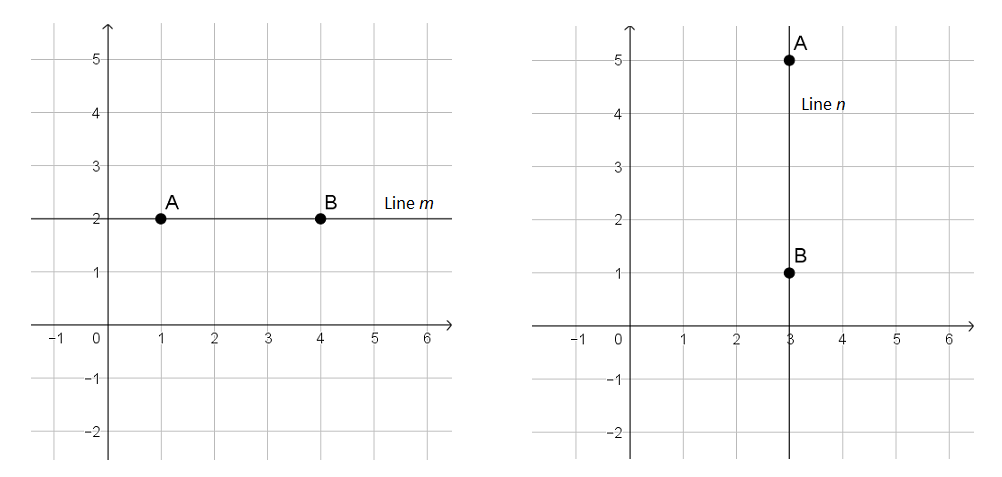# Linear Relationships

## Objective

Determine slope from coordinate points. Find slope of horizontal and vertical lines.

## Common Core Standards

### Core Standards

?

• 8.EE.B.6 — Use similar triangles to explain why the slope m is the same between any two distinct points on a non-vertical line in the coordinate plane; derive the equation y = mx for a line through the origin and the equation y = mx + b for a line intercepting the vertical axis at b.

• 8.F.B.4 — Construct a function to model a linear relationship between two quantities. Determine the rate of change and initial value of the function from a description of a relationship or from two (x, y) values, including reading these from a table or from a graph. Interpret the rate of change and initial value of a linear function in terms of the situation it models, and in terms of its graph or a table of values.

?

• 7.NS.A.1

• 7.NS.A.2.B

## Criteria for Success

?

1. Find the slope of a line using values represented in a table.
2. Find the slope of a line that passes through two given coordinate points.
3. Understand the slope of a horizontal line is 0 and the slope of a vertical line is undefined.

## Tips for Teachers

?

In determining slope from coordinate points, students will need a strong grasp of adding and subtracting signed numbers (standard 7.NS.1). If needed, review these concepts and skills prior to this lesson.

### Remote Learning Guidance

If you need to adapt or shorten this lesson for remote learning, we suggest prioritizing Anchor Problems 2 and 3 (benefit from worked examples). Find more guidance on adapting our math curriculum for remote learning here.

#### Fishtank Plus

Subscribe to Fishtank Plus to unlock access to additional resources for this lesson, including:

• Problem Set
• Student Handout Editor
• Google Classrom Integration
• Vocabulary Package

## Anchor Problems

?

### Problem 1

The table below shows some solution values for the equation ${{y=-3x+2}}$.

 $x$ $y$ -2 8 -1 5 0 2 1 -1

Use the values in the table to determine the slope of the line represented by ${{y=-3x+2}}$.

#### Guiding Questions

Create a free account or sign in to access the Guiding Questions for this Anchor Problem.

### Problem 2

Find the slope of the line between the points (-1, 3) and (5, 11).

Is the point (-4, -1) on the same line as the other two points? Use slope to justify your answer.

#### Guiding Questions

Create a free account or sign in to access the Guiding Questions for this Anchor Problem.

### Problem 3

Find the slope of line $m$ and line $n$, shown below.#### Guiding Questions

Create a free account or sign in to access the Guiding Questions for this Anchor Problem.

## Problem Set

?With Fishtank Plus, you can download a complete problem set and answer key for this lesson. Download Sample

The following resources include problems and activities aligned to the objective of the lesson that can be used to create your own problem set.

• Challenge: Line $k$ has a slope of $-\frac{1}{2}$. It passes through the points $(x, -2)$ and $(-3, \frac{1}{4})$. Find the value of $x$ in the coordinate point.

## Target Task

?

Samantha found the slope of the line that passed through the points ${(2, 6)}$ and ${(-4, 8)}$. Her work is shown below.

${{8-6\over {2-(-4)}} = {2\over 2+4 }= {2\over 6} = {1\over3}}$

Samantha made an error in her work. Describe the error and then find the correct slope of the line through the two given points.

Create a free account or sign in to view Mastery Response